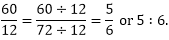×#### Thank you for registering.

One of our academic counsellors will contact you within 1 working day.

Click to Chat

1800-1023-196

+91-120-4616500

CART 0

• 0

MY CART (5)

Use Coupon: CART20 and get 20% off on all online Study Material

ITEM
DETAILS
MRP
DISCOUNT
FINAL PRICE
Total Price: Rs.

There are no items in this cart.
Continue Shopping• Complete JEE Main/Advanced Course and Test Series
• OFFERED PRICE: Rs. 15,900
• View Details

```Chapter 9: Ratio, Proportion and Unitary Method Exercise 9.1

Ratio, Proportion and Unitary Method – Exercise 9.1 – Q.1

(i) Ratio of number of girls of girls to that of boys in the merit list is 2 : 1.

(ii) Ratio of number of students passing a mathematics test to that of total students appearins in test is 2 : 3.

Ratio, Proportion and Unitary Method – Exercise 9.1 – Q.2

(i) The number of bad pencils produced in a factory is 1/9 of the number of good pencils produced in the factory.

(ii) The number of villages is 2000 times that of cities in India.

Ratio, Proportion and Unitary Method – Exercise 9.1 – Q.3

(i) 60 : 72.

To express this ratio in the simplest form, we will have to find the H.C.F of 60 and 72.

It is 12 dividing each term of the ration by the H.C.F of its terms i.e. 12 we getHence, the simplest form of the ratio 60: 72 is 5 : 6.

(ii) 324: 144

To express this ratio in the simplest form, we will have to find the H.C.F of 324/144, It is 36.Dividing each term of the ration by the H.C.F of its terms i.e. 36, we getHence, the simplest form of the ratio 324: 144 is 9:4.

(iii) 85 : 391.

To express this ratio in the simplest form we will have to find the H.C.F of 85 and 391, It is 17.

Dividing each term of the ratio by the H.C.F of its terms i.e. 17, we getHence, the simplest form of the ratio 85:391 is 5:23

(iv) 186 : 403.

The given ratio is 186 : 403 = 186/403

To express this ratio in the simplest form, we will have to find the H.C.F of 186 and 403, It is 31.

Dividing each term of the ratio by the H.C.F of its terms i.e. 31, we get 186: 403 is 6:23.

Ratio, Proportion and Unitary Method – Exercise 9.1 – Q.4

(i) 75 paise to Rs 3 = 75 paise : Rs 3

= 75 paise : 300 paise

= 1 : 4.

[∵ IRS = 100 paise]

[Dividing the first and second term by their H.C.R = 75]

(ii) 35 minutes to 45 minutes

= 35 min : 45 min

= 7 : 9 [diving the first-and second term by their H.C.F = 5]

(iii) 8kg to 400 gm. = 8 kg : 400 gm

= 8000 gm : 400 gm

= 20 : 1.

[dividing the first and second terms by their H.C.F = 400]

(iv) 48 minutes to 1 hour = 48 min: 1 hour

= 48 min: 60 min

[∵ 1 hour = 60 min] = 4: 5.

[Dividing the first and second term by their H.C.F = 12]

(v) 2 meters to 35 cm = 2 met: 35 cm

= 200 cm : 35 cm

[1m = 100 cm]

= 40 : 7

[∵ dividing the first and second term by their H.C.F = 5].

[∵ dividng the first and second term by their H.C.F = 5]

(vi) 35 minutes to 45 seconds = 35 min : 45 sec

= 2100 sec: 45 sec

= 140:3 [H.C.F = 15]

(vii) 2 dozen to 3 scores = 2 dozen : 3 scores

= 24: 60

[∵ 1 dozen = 12 score = 20] = 2:5.

[Dividing the first and second term by their H.C.F = 12].

(viii) 3 weeks to 3 days = 3 weeks : 3 days

= 21 day: 3days

[1 week = 7 days]

= 3 × 7 = 3

= 7:1.

(ix) 48 min to 2 hours 40 min = 48 min : 160 min

[∵ 1 hour = 60 min]

= 3: 10

[∵ dividing the first and second term by their H.C.F = 3:10]

(x) 3m 5cm to 35 cm = 3m 5 cm : 35 cm

= 305 cm : 35 cm

[dividing the first and second terms by their H.C.F = 5] = 61 : 7
```### Course Features

• 728 Video Lectures
• Revision Notes
• Previous Year Papers
• Mind Map
• Study Planner
• NCERT Solutions
• Discussion Forum
• Test paper with Video Solution